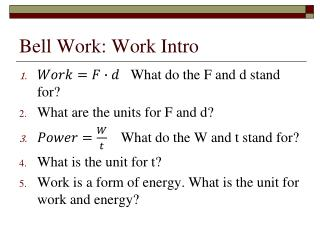DownloadDownload PresentationBell Work: Work Intro

# Bell Work: Work Intro

Télécharger la présentation## Bell Work: Work Intro

- - - - - - - - - - - - - - - - - - - - - - - - - - - E N D - - - - - - - - - - - - - - - - - - - - - - - - - - -
##### Presentation Transcript

1. Bell Work: Work Intro • What do the F and d stand for? • What are the units for F and d? • What do the W and t stand for? • What is the unit for t? • Work is a form of energy. What is the unit for work and energy?

2. Work and Power

3. Work • Work is a force causing something to move a distance. • Work = force x distance • W=f x d • Units for work: Newton-meter (N-m) or Joule • Direction of motion and force must be the same.

4. Work is NOT • Work does not involve time. • Work is not motionless.

5. Examples • Example of work: • weight lifter pushing barbells from shoulder to over head • Two men moving 20 boxes to another room. • Example of NO work: • weight lifter holding barbells over his head • Two men taking a break from moving boxes

6. Power • Power - how fast work is done. • Power is the rate at which work is done, or amount of work done per unit of time. • Power = work divided by time P = W/t

7. Power Units • Units for power: Newton-meter/second (N-m/s) or Joule/second (J/sec) or Watt (W) • Kilowatt (kW) – used to measure large quantities of power like electrical power. • 1000 W in a kW • Horsepower (hp) – also a unit of power. 1 hp = 750 watts

8. Examples • Lifting barbell in 2 seconds • Two men moving 20 boxes to another room in 10 minutes.

9. Machines • A machine is a device that makes work easier. • Examples: hammer, bicycle ramp, scissors, shovel, and doorknob

10. Machines • Machines can do a variety of jobs: pump water from a well, hoist a sail, plow a field, catch a fish. • Machines make work easier by changing the size or direction of the applied force.

11. Two forces involved in using a machine 1. The force applied to a machine is the effort force (FE) • Example: when you pull down on the handle of a crowbar, you are applying an effort force.

12. Two forces… 2. The force applied by the machine is the resistance force (FR) • Example: Motor turns fan

13. Input • Work input (WI) – work done on a machine • WI = FE x dE • Example: work input of the crowbar (WI) is equal to the force you apply to the handle (FE) times the distance the handle moves (dE)

14. Output • Work Output –Work done by a machine . • WO = FR x dR • Example: The work output of the crowbar (WO) is equal to the force the crowbar exerts on the nail (FR ) times the distance the nail moves (dR).

15. What do machines do?? • Machines make work easier, but do not multiply work. • So, work output can never be greater than work input

16. Mechanical Advantage • The number of times a machine multiplies the effort force is called the mechanical advantage (MA) • MA = FR/FE • Example: if a crowbar allows you to exert only 20 newtons of force to raise a 200 newton object, its mechanical advantage is 10.

17. Efficiency • The comparison of work output to work input is called the efficiency of a machine. • Efficiency = WO/WI x 100 • High efficiency means that much of the work input is changed to useful work output. • Low efficiency means that much of the work input is lost and a great deal of useful work output does not result.

18. Simple Machines I. Inclined Plane – a slanted surface used to raise an object. Example: a ramp • Wedges and Screws – wedge is an inclined plane that moves. Have an edge that is thinner at one end. Examples: knife, ax, wedge • A screw is also an inclined plane. It is an inclined plane wrapped around a cylinder to form a spiral.

19. Simple Machines II. Levers – a bar that is free to pivot a fixed point when an effort force is applied. The fixed point of the pivot is called the fulcrum.

20. Levers ****Three classes of levers: • Fulcrum is between the effort force and the resistance force. Example: crowbar, pliers, scissors and seesaws • Resistance force is between fulcrum and effort force. Examples: wheelbarrows, doors • Effort force is greater than the resistance force and the fulcrum

21. Simple machines III. Pulleys – a chain, belt, or rope wrapped around a wheel. A pulley can change either the direction or the amount of an effort force. IV. Wheel and Axle – a lever that rotates in a circle. Made of 2 wheels of different sizes. Examples: bicycles, Ferris wheels, water wheels and gears.